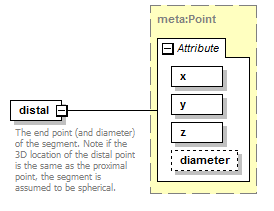# distal

 `The end point (and diameter) of the segment. Note if the 3D location of the distal point is the same as the proximal point, the segment is assumed to be spherical.`

## Model## Attributes

QName Type Fixed Default Use Inheritable Annotation
diameter xs:double optional
x xs:double required
y xs:double required
z xs:double required

## Source

 ``` The end point (and diameter) of the segment. Note if the 3D location of the distal point is the same as the proximal point, the segment is assumed to be spherical. ```